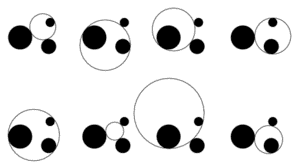A# Apollonius problemApollonius circles.

The Apollonius problem is: given three objects in the plane, each of which may be a circle C, a point P (a degenerate circle), or a line L (part of a circle with infinite radius), find another circle that is tangent to (just touches) each of the three. This problem was first recorded in Tangencies, written around 200 BC by Apollonius of Perga and so is named after him.

There are ten cases: PPP, PPL, PLL, LLL, PPC, PLC, LLC, LCC, PCC, CCC. The two easiest involve three points or three straight lines and were first solved by Euclid. Solutions to the eight other cases, with the exception of the three-circle problem, appeared in Tangencies; however, this work was lost. The most difficult case, to find a tangent circle to any three other circles, was first solved by the French mathematician François Viète (1540–1603) and involves the simultaneous solution of three quadratic equations, although, in principle, a solution could be found using just a compass and a straightedge.

Any of the eight circles that is a solution to the general three-circle problem is called an Apollonius circle. If the three circles are mutually tangent then the eight solutions collapse to just two, which are known as Soddy circles. If, having started with three mutually tangent circles and having created a fourth – the inner Soddy circle – that is nested between the original three, the process is repeated to yield three more circles nested between sets of three of these, and then repeated again indefinitely, a fractal is produced. The points that are never inside a circle form a fractal set called the Apollonian gasket, which has a fractional dimension of about 1.30568.